Quantile g-computation (qgcomp) is a special case of g-computation used for estimating joint exposure response curves for a set of continuous exposures. The base package qgcomp allows one to estimate conditional or marginal joint-exposure response curves. Because this approach developed within the field of “exposure mixtures” the set of exposures of interest are referred to here as “the mixture.” qgcompint builds on qgcomp by incorporating statistical interaction (product terms) between binary, categorical, or continuous covariates and the mixture.

The model

Say we have an outcome $$Y$$, some exposures $$\mathbb{X}$$, a “modifier” or a covariate for which we wish to assess statistical interaction with $$\mathbb{X}$$, denoted by $$M$$ and possibly some other covariates (e.g. potential confounders) denoted by $$\mathbb{Z}$$.

The basic model of quantile g-computation is a joint marginal structural model given by

$\mathbb{E}(Y^{\mathbf{X}_q} | M, \mathbf{Z,\psi,\eta}) = g(\psi_0 + \psi_1 S_q + \psi_2 M + \psi_3 M\times S_q + \mathbf{\eta Z})$

where $$g(\cdot)$$ is a link function in a generalized linear model (e.g. the inverse logit function in the case of a logistic model for the probability that $$Y=1$$), $$\psi_0$$ is the model intercept, $$\mathbf{\eta}$$ is a set of model coefficients for the covariates and $$S_q$$ is an “index” that represents a joint value of exposures. The joint exposure has a “main effect” at the referent value of $$M$$ given by $$\psi_1$$, $$\psi_2$$ represents the association (or set of associations for categorical $$M$$) between the modifier and the outcome, and $$\psi_3$$ is a product terms (or set of product terms for categorical $$M$$) that represent the deviation of the exposure response for $$S_q$$ from the main effect for each one unit increase in $$M$$. The magnitude of $$\psi_3$$ can be used to estimate the extent of statistical interaction on the model scale, sometimes referred to as effect measure modification.

Quantile g-computation (by default) transforms all exposures $$\mathbf{X}$$ into $$\mathbf{X}_q$$, which are “scores” taking on discrete values 0,1,2,etc. representing a categorical “bin” of exposure. By default, there are four bins with evenly spaced quantile cutpoints for each exposure, so $${X}_q=0$$ means that $$X$$ was below the observed 25th percentile for that exposure. The index $$S_q$$ represents all exposures being set to the same value (again, by default, discrete values 0,1,2,3). Thus, the parameter $$\psi_1$$ quantifies the expected change in the outcome, given a one quantile increase in all exposures simultaneously, possibly adjusted for $$\mathbf{Z}$$.

There are nuances to this particular model form that are available in the qgcompint package which will be explored below. There exists one special case of quantile g-computation that leads to fast fitting: linear/additive exposure effects. Here we simulate “pre-quantized” data where the exposures $$X_1, X_2, …, X_7$$ can only take on values of 0,1,2,3 in equal proportions. The model underlying the outcomes is given by the linear regression:

$\mathbb{E}(Y | \mathbf{X}, M,\beta,\psi,\eta) = \beta_0 + \beta_1 X_1 + … + \beta_7 X_7 + \psi_2 M +\eta_9 X_1\times M, …, +\eta_{15} X_7\times M$

with the true values of $$\beta$$ given by:

##        value
## psi_0    0.0
## beta_1   0.8
## beta_2   0.6
## beta_3   0.3
## beta_4  -0.3
## beta_5  -0.3
## beta_6  -0.3
## beta_7   0.0
## psi_2    0.0
## eta_1    1.0
## eta_2    0.0
## eta_3    0.0
## eta_4    0.0
## eta_5    0.2
## eta_6    0.2
## eta_7    0.2

In this example $$X_1$$ is positively correlated with $$X_2-X_4$$ ($$\rho=0.8,0.6,0.3$$) and negatively correlated with $$X_5-X_7$$ ($$\rho=-0.3-0.3,-0.3$$). In this setting, the parameter $$\psi_1$$ will equal the sum of the $$\beta_1-\beta_7$$ coefficients (0.8), $$\psi_2$$ is given directly by the data generation model (0.0), and $$\psi_3$$ will equal the sum of the $$\eta$$ coefficients (1.6). Simulating data to fit this model is available within a the simdata_quantized_emm function in the qgcompint package. Here, we simulate data using a binary modifier and inspect the correlation matrix to see that the estimated correlation matrix is approximately the same as the correlation of the data generation mechanism. These will converge in large sample sizes, but for a sample size of 200, the estimated coefficients will differ from those we simulate under due to random variation (set the sample size to 10000 in this example to confirm).

library(qgcompint)
set.seed(42)
dat1 <- simdata_quantized_emm(
outcometype="continuous",
# sample size
n = 300,
# correlation between x1 and x2,x3,...
corr=c(0.8,0.6,0.3,-0.3,-0.3,-0.3),
# model intercept
b0=0,
# linear model coefficients for x1,x2,... at referent level of interacting variable
mainterms=c(0.3,-0.1,0.1,0.0,0.3,0.1,0.1),
# linear model coefficients for product terms between x1,x2,... and interacting variable
prodterms = c(1.0,0.0,0.0,0.0,0.2,0.2,0.2),
# type of interacting variable
ztype = "binary",
# number of levels of exposure
q = 4,
# residual variance of y
yscale = 2.0
)
names(dat1)[which(names(dat1)=="z")] = "M"

print("data")
##  "data"
##   M x1 x2 x3 x4 x5 x6 x7        y
## 1 1  0  1  0  0  2  3  3 3.231033
## 2 1  0  3  0  0  3  2  1 1.685041
## 3 0  1  1  1  0  2  1  2 2.459104
## 4 1  2  2  0  2  3  1  1 4.750329
## 5 1  1  1  3  1  3  1  3 2.756988
## 6 1  1  0  1  1  3  0  2 4.711521
print("modifier")
##  "modifier"
table(dat1$M) ## ## 0 1 ## 151 149 print("outcome") ##  "outcome" summary(dat1$y)
##    Min. 1st Qu.  Median    Mean 3rd Qu.    Max.
## -4.9302  0.7028  2.4380  2.5628  4.2134 10.0813
print("exposure correlation")
##  "exposure correlation"
cor(dat1[,paste0("x",1:7)])
##            x1         x2         x3          x4          x5          x6
## x1  1.0000000  0.8240000  0.6213333  0.33866667 -0.34666667 -0.28266667
## x2  0.8240000  1.0000000  0.4800000  0.33600000 -0.32533333 -0.23200000
## x3  0.6213333  0.4800000  1.0000000  0.26133333 -0.20800000 -0.23733333
## x4  0.3386667  0.3360000  0.2613333  1.00000000 -0.12800000 -0.03200000
## x5 -0.3466667 -0.3253333 -0.2080000 -0.12800000  1.00000000  0.09066667
## x6 -0.2826667 -0.2320000 -0.2373333 -0.03200000  0.09066667  1.00000000
## x7 -0.3653333 -0.3626667 -0.2346667 -0.04533333  0.16800000  0.12000000
##             x7
## x1 -0.36533333
## x2 -0.36266667
## x3 -0.23466667
## x4 -0.04533333
## x5  0.16800000
## x6  0.12000000
## x7  1.00000000

fitting a model with a modifier

Here we see that qgcomp (via the function qgcomp.emm.noboot) estimates a $$\psi_1$$ fairly close to 0.8 (estimate = 0.7) (again, as we increase sample size, the estimated value will be expected to become increasingly close to the true value). The product term 'M:mixture' is the $$\psi_3$$ parameter noted above, which is also fairly close to the true value of 1.6 (estimate = 1.7).

For binary modifiers, qgcomp.emm.noboot will also estimate the joint effect of the mixture in strata of the modifier. Here, the effect of the mixture at $$M=0$$ is given by $$\psi_1$$, whereas the effect of the mixture at $$M=1$$ is estimated below the coefficient table (and is here given by $$\psi_1+\psi_3$$ = 2.4).

qfit1 <- qgcomp.emm.noboot(y~x1+x2+x3+x4+x5+x6+x7,
data = dat1,
expnms = paste0("x",1:7),
emmvar = "M",
q = 4)
qfit1
## ## Qgcomp weights/partial effects at M = 0
## Scaled effect size (positive direction, sum of positive effects = 1.05)
##    x4    x1    x5    x7
## 0.393 0.237 0.219 0.151
## Scaled effect size (negative direction, sum of negative effects = -0.376)
##    x6    x3    x2
## 0.449 0.435 0.116
##
##
## ## Qgcomp weights/partial effects at M = 1
## Scaled effect size (positive direction, sum of positive effects = 2.89)
##     x1     x5     x6     x7     x3
## 0.5930 0.1879 0.1049 0.0839 0.0303
## Scaled effect size (negative direction, sum of negative effects = -0.535)
##  x4  x2
## 0.7 0.3
##
##
## ## Mixture slope parameters (Delta method CI):
##
##             Estimate Std. Error  Lower CI Upper CI t value Pr(>|t|)
## (Intercept)  0.14559    0.61921 -1.068034   1.3592  0.2351  0.81428
## psi1         0.67542    0.39146 -0.091832   1.4427  1.7254  0.08555
## M            0.36067    0.87113 -1.346720   2.0681  0.4140  0.67917
## M:mixture    1.68390    0.56052  0.585307   2.7825  3.0042  0.00290
##
## Estimate (CI), M=1:
## 2.3593 (1.573, 3.1456)

getting bounds for pointwise comparisons

As in qgcomp you can estimate pointwise comparisons along the joint regression line. Here we estimate them at both values of $$M$$ (via the emmval parameter).

pointwisebound(qfit1, emmval=0)
##   quantile quantile.midpoint        hx mean.diff   se.diff     ll.diff  ul.diff
## 1        0             0.125 0.1455920 0.0000000 0.0000000  0.00000000 0.000000
## 2        1             0.375 0.8210108 0.6754188 0.3914617 -0.09183203 1.442670
## 3        2             0.625 1.4964296 1.3508376 0.7829234 -0.18366407 2.885339
## 4        3             0.875 2.1718484 2.0262564 1.1743851 -0.27549610 4.328009
##   emm_level
## 1         0
## 2         0
## 3         0
## 4         0
pointwisebound(qfit1, emmval=1)
##   quantile quantile.midpoint        hx mean.diff   se.diff  ll.diff  ul.diff
## 1        0             0.125 0.5062631  0.000000 0.0000000 0.000000 0.000000
## 2        1             0.375 2.8655840  2.359321 0.4011708 1.573041 3.145601
## 3        2             0.625 5.2249050  4.718642 0.8023416 3.146081 6.291203
## 4        3             0.875 7.5842259  7.077963 1.2035125 4.719122 9.436804
##   emm_level
## 1         1
## 2         1
## 3         1
## 4         1

plotting weights (weights are at referent level of modifier)

For qgcomp.emm.noboot fits, a set of “weights” will be given that are interpreted as the proportion of a “partial” effect for each variable. That is, $$\psi_1$$ will represent the joint effect of multiple exposures, some of which will have independent effects that are positive, and some will have negative independent effects. For example, the “negative partial effect” is simply the sum of all of the negative independent effects (this is only given for a model in which all exposures are included via linear terms and no interactions among exposures occur). These weights are conditional on the fitted model, and so are not “estimates” per se and will not have associated confidence intervals. Nonetheless, the weights are useful for interpretation of the joint effect.

Notably, with product terms in the model for the joint effect, a different set of weights will be generated at every value of the modifier. Here, we can plot the weights at M=0 and M=1.

plot(qfit1, emmval=0)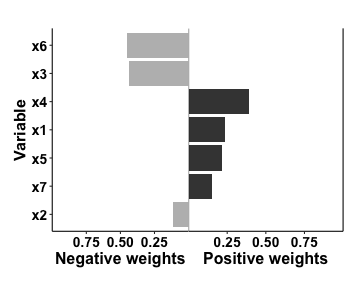plot(qfit1, emmval=1)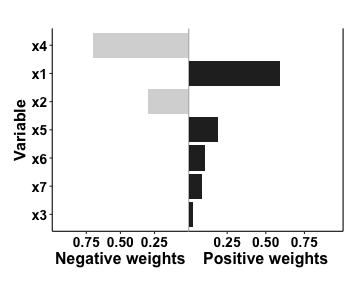bootstrapping

For non-linear qgcomp fits, or to get marginal estimates of the exposure-response curve (i.e. conditional only on the modifier), we can use the qgcomp.emm.boot function. Here we just repeat the original fit, which yields similar evidence that there is substantial statistical interaction on the additive scale, so that we would expect the joint exposure effect estimate to be greater for M=1 than for M=0, which corroborates the fit above (the point estimates are identical, as expected in this case due to no non-modifier covariates, so this is not a surprise).

qfit1b <- qgcomp.emm.boot(y~x1+x2+x3+x4+x5+x6+x7,
data=dat1,
expnms = paste0("x",1:7),
emmvar = "M",
q = 4)
qfit1b
## ## Mixture slope parameters (bootstrap CI):
##
##             Estimate Std. Error  Lower CI Upper CI t value Pr(>|t|)
## (Intercept)  0.14559    0.60441 -1.039024   1.3302  0.2409 0.809819
## psi1         0.67542    0.38573 -0.080604   1.4314  1.7510 0.081029
## M            0.36067    0.81015 -1.227190   1.9485  0.4452 0.656522
## M:mixture    1.68390    0.52371  0.657445   2.7104  3.2153 0.001454

plotting

plot(qfit1b, emmval=0)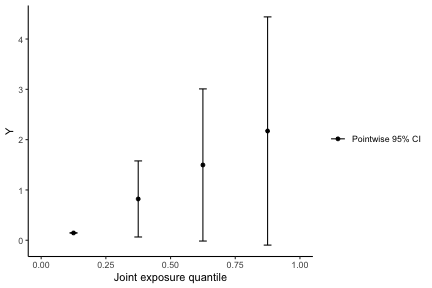plot(qfit1b, emmval=1)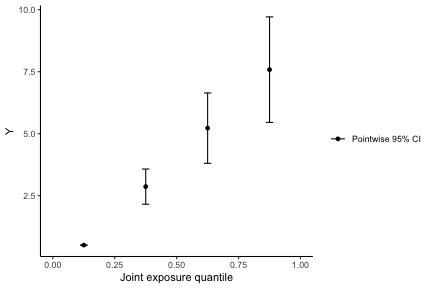plotting with same y axis

Visually, it's easier to compare two plots with the same y-axis. Here we can see that the joint regression curve is steeper at M=1 than it is at M=0

p1 <- plot(qfit1b, emmval=0, suppressprint = TRUE)
p2 <- plot(qfit1b, emmval=1, suppressprint = TRUE)
p1 + ggplot2::coord_cartesian(ylim=c(0,10))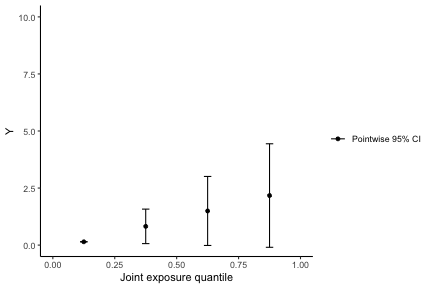p2 + ggplot2::coord_cartesian(ylim=c(0,10))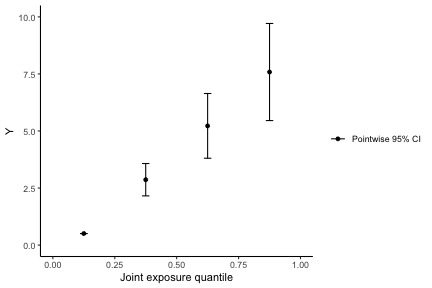categorical modifier, binary outcome

Now we can simulate data under a categorical modifier, and the simdata_quantized_emm function will pick some convenient defaults. We will also use a binary outcome with N=300 to allow that use of a categorical modifier with a rare binary outcome will be subject to low power and potential for bootstrapping to fail due to empty strata in some bootstrap samples.

Simulated data defaults

Here is a good place to note that simdata_quantized_emm is provided mainly as a learning tool. While it could potentially be used for simulations in a scientific publication, it likely has too many defaults that are not user controllable that may be useful to be able to change. Some of the underlying code that may serve as inspiration for more comprehensive simulations can be explored via the “hidden” (non-exported) functions qgcompint:::.quantized_design_emm,qgcompint:::.dgm_quantized_linear_emm, qgcompint:::.dgm_quantized_logistic_emm, and qgcompint:::.dgm_quantized_survival_emm.

set.seed(23)
dat2 <- simdata_quantized_emm(
outcometype="logistic",
# sample size
n = 300,
# correlation between x1 and x2,x3,...
corr=c(0.6,0.5,0.3,-0.3,-0.3,0.0),
# model intercept
b0=-2,
# linear model coefficients for x1,x2,... at referent level of interacting variable
mainterms=c(0.1,-0.1,0.1,0.0,0.1,0.1,0.1),
# linear model coefficients for product terms between x1,x2,... and interacting variable
prodterms = c(0.2,0.0,0.0,0.0,0.2,-0.2,0.2),
# type of interacting variable
ztype = "categorical",
# number of levels of exposure
q = 4,
# residual variance of y
yscale = 2.0
)

print("data")
##  "data"
##   z x1 x2 x3 x4 x5 x6 x7 y
## 1 0  2  2  2  2  3  0  3 0
## 2 2  0  2  0  3  1  3  3 0
## 3 0  3  0  3  1  0  3  0 0
## 4 2  2  2  2  3  1  1  0 0
## 5 2  3  3  0  3  0  1  1 0
## 6 0  3  0  3  1  3  1  3 0
print("modifier")
##  "modifier"
table(dat2$z) ## ## 0 1 2 ## 109 92 99 print("outcome") ##  "outcome" table(dat2$y)
##
##   0   1
## 206  94
print("exposure correlation")
##  "exposure correlation"
cor(dat2[,paste0("x",1:7)])
##             x1          x2         x3           x4           x5           x6
## x1  1.00000000  0.53866667  0.5493333  0.210666667 -0.272000000 -0.285333333
## x2  0.53866667  1.00000000  0.2693333  0.186666667 -0.130666667 -0.205333333
## x3  0.54933333  0.26933333  1.0000000  0.109333333 -0.186666667 -0.184000000
## x4  0.21066667  0.18666667  0.1093333  1.000000000 -0.069333333 -0.005333333
## x5 -0.27200000 -0.13066667 -0.1866667 -0.069333333  1.000000000  0.005333333
## x6 -0.28533333 -0.20533333 -0.1840000 -0.005333333  0.005333333  1.000000000
## x7 -0.01066667 -0.05066667 -0.0480000  0.029333333  0.029333333  0.002666667
##              x7
## x1 -0.010666667
## x2 -0.050666667
## x3 -0.048000000
## x4  0.029333333
## x5  0.029333333
## x6  0.002666667
## x7  1.000000000

wrong way to fit

Below is one way to fit qgcomp.emm.noboot with a categorical modifier that exactly follows the previous code. This approach to categorical modifiers is incorrect, in this case, due to the format of these data. Note if you fit the model like this, where your categorical modifier is not the proper data type, qgcomp.emm.noboot will assume you have a continuous modifier.

qfit.wrong <- qgcomp.emm.noboot(y~x1+x2+x3+x4+x5+x6+x7,
data = dat2,
expnms = paste0("x",1:7),
emmvar = "z",
q = 4, family=binomial())
qfit.wrong
## ## Qgcomp weights/partial effects at z = 0
## Scaled effect size (positive direction, sum of positive effects = 1.11)
##     x5     x3     x1     x6
## 0.3770 0.2755 0.2614 0.0862
## Scaled effect size (negative direction, sum of negative effects = -0.228)
##    x2    x4    x7
## 0.694 0.207 0.099
##
##
## ## Mixture log(OR) (Delta method CI):
##
##             Estimate Std. Error Lower CI Upper CI Z value Pr(>|z|)
## (Intercept) -2.72213    0.92249 -4.53018 -0.91408 -2.9509 0.003169
## psi1         0.88437    0.55971 -0.21263  1.98137  1.5801 0.114093
## z           -0.41716    0.71710 -1.82266  0.98834 -0.5817 0.560751
## z:mixture    0.62691    0.44231 -0.24001  1.49382  1.4173 0.156383

The right way to fit with categorical modifier (use as.factor())

Instead, you should convert each categorical modifier to a “factor” prior to fitting the model. Here you can see the output for both the non-bootstrapped fit and the fit with bootstrapped confidence intervals (since there are no other covariates in the model, these two approaches estimate the same marginal effect and parameter log-odds ratio estimates will be identical). Here we see that we get a unique interaction term and main effect for each level of the modifier z.

dat2$zfactor = as.factor(dat2$z)
# using asymptotic-based confidence intervals
qfit2 <- qgcomp.emm.noboot(y~x1+x2+x3+x4+x5+x6+x7,
data = dat2,
expnms = paste0("x",1:7),
emmvar = "zfactor",
q = 4, family=binomial())
# using bootstrap based confidence intervals (estimate a)
set.seed(12312)
qfit2b <- qgcomp.emm.boot(y~x1+x2+x3+x4+x5+x6+x7,
data = dat2,
expnms = paste0("x",1:7),
emmvar = "zfactor",
q = 4, family=binomial(), rr = FALSE)
qfit2
## ## Qgcomp weights/partial effects at zfactor = 0
## Scaled effect size (positive direction, sum of positive effects = 1.15)
##     x5     x3     x1     x6
## 0.3914 0.3857 0.2014 0.0215
## Scaled effect size (negative direction, sum of negative effects = -0.382)
##    x7    x4    x2
## 0.427 0.351 0.222
##
##
## ## Mixture log(OR) (Delta method CI):
##
##                  Estimate Std. Error Lower CI Upper CI Z value Pr(>|z|)
## (Intercept)      -2.80404    1.08650 -4.93355 -0.67454 -2.5808 0.009857
## psi1              0.76337    0.65463 -0.51969  2.04642  1.1661 0.243576
## zfactor1         -0.89742    1.55815 -3.95134  2.15651 -0.5759 0.564650
## zfactor1:mixture  1.46319    0.97182 -0.44155  3.36793  1.5056 0.132166
## zfactor2         -0.49507    1.48536 -3.40631  2.41617 -0.3333 0.738908
## zfactor2:mixture  1.02544    0.90842 -0.75503  2.80591  1.1288 0.258974
qfit2b
## ## Mixture log(OR) (bootstrap CI):
##
##                  Estimate Std. Error Lower CI Upper CI Z value Pr(>|z|)
## (Intercept)      -2.80404    1.26438 -5.28218 -0.32591 -2.2177  0.02657
## psi1              0.76337    0.77474 -0.75510  2.28183  0.9853  0.32447
## zfactor1         -0.89742    1.75495 -4.33706  2.54223 -0.5114  0.60910
## zfactor2         -0.49507    1.69782 -3.82274  2.83261 -0.2916  0.77060
## zfactor1:mixture  1.46319    1.08689 -0.66707  3.59345  1.3462  0.17823
## zfactor2:mixture  1.02544    1.05214 -1.03671  3.08759  0.9746  0.32974

getting bounds for pointwise comparisons

Here are some miscellaneous functions for getting point estimates and bounds for various comparisons at specific values of the modifier.

print("output the weights at Z=0")
##  "output the weights at Z=0"
getstratweights(qfit2, emmval=0)
## ## Qgcomp weights/partial effects at zfactor = 0
## Scaled effect size (positive direction, sum of positive effects = 1.15)
##     x5     x3     x1     x6
## 0.3914 0.3857 0.2014 0.0215
## Scaled effect size (negative direction, sum of negative effects = -0.382)
##    x7    x4    x2
## 0.427 0.351 0.222
print("output pointwise comparisons at Z=0")
##  "output pointwise comparisons at Z=0"
pointwisebound(qfit2, emmval=0)
##   quantile quantile.midpoint         hx       or   se.lnor     ll.or      ul.or
## 1        0             0.125 -2.8040444 1.000000 0.0000000 1.0000000   1.000000
## 2        1             0.375 -2.0406788 2.145485 0.6546337 0.5947033   7.740173
## 3        2             0.625 -1.2773131 4.603106 1.3092673 0.3536720  59.910280
## 4        3             0.875 -0.5139474 9.875896 1.9639010 0.2103299 463.715942
##   emm_level
## 1         0
## 2         0
## 3         0
## 4         0
print("plot weights at Z=0")
##  "plot weights at Z=0"
plot(qfit2, emmval=0)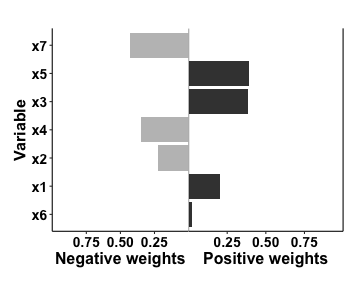print("output stratum specific joint effect estimate for the mixture at Z=2")
##  "output stratum specific joint effect estimate for the mixture at Z=2"
print(getstrateffects(qfit2, emmval=2))
## Joint effect at zfactor=2
##         Estimate Std. Error Lower CI Upper CI z value Pr(>|z|)
## Mixture  1.78881    0.62983  0.55437   3.0232  2.8402 0.004509
print("output the weights at Z=2")
##  "output the weights at Z=2"
print(getstratweights(qfit2, emmval=2))
## ## Qgcomp weights/partial effects at zfactor = 2
## Scaled effect size (positive direction, sum of positive effects = 1.9)
##    x5    x1    x3    x2    x4
## 0.426 0.293 0.136 0.110 0.035
## Scaled effect size (negative direction, sum of negative effects = -0.116)
##    x6    x7
## 0.835 0.165
print("output pointwise comparisons at Z=2")
##  "output pointwise comparisons at Z=2"
pointwisebound(qfit2, emmval=2)
##   quantile quantile.midpoint         hx         or   se.lnor    ll.or
## 1        0             0.125 -3.2991126   1.000000 0.0000000 1.000000
## 2        1             0.375 -1.5103038   5.982322 0.6298277 1.740843
## 3        2             0.625  0.2785049  35.788174 1.2596553 3.030533
## 4        3             0.875  2.0673137 214.096371 1.8894830 5.275680
##        ul.or emm_level
## 1    1.00000         2
## 2   20.55796         2
## 3  422.62977         2
## 4 8688.40648         2
plot(qfit2, emmval=2)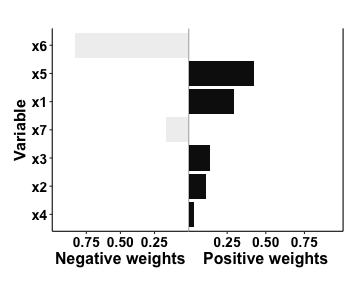print("output stratum specific joint effect estimate for the mixture at Z=2 from bootstrapped fit")
##  "output stratum specific joint effect estimate for the mixture at Z=2 from bootstrapped fit"
print(getstrateffects(qfit2b, emmval=2))
## Joint effect at zfactor=2
##         Estimate Std. Error Lower CI Upper CI z value Pr(>|z|)
## Mixture  1.78881    0.62983  0.55437   3.0232  2.8402 0.004509
print("output pointwise comparisons at Z=2 from bootstrapped fit")
##  "output pointwise comparisons at Z=2 from bootstrapped fit"
print(pointwisebound(qfit2b, emmval=2))
##   quantile quantile.midpoint         hx         or   se.lnor    ll.or
## 1        0             0.125 -3.2991126   1.000000 0.0000000 1.000000
## 2        1             0.375 -1.5103038   5.982322 0.7467761 1.384245
## 3        2             0.625  0.2785049  35.788174 1.4935522 1.916133
## 4        3             0.875  2.0673137 214.096371 2.2403283 2.652396
##         ul.or ll.linpred  ul.linpred emm_level
## 1     1.00000  -3.299113 -3.29911257         2
## 2    25.85394  -2.973958 -0.04664959         2
## 3   668.42618  -2.648804  3.20581339         2
## 4 17281.44980  -2.323649  6.45827637         2
print("output modelwise confidence bounds at Z=2 from bootstrapped fit")
##  "output modelwise confidence bounds at Z=2 from bootstrapped fit"
print(modelbound(qfit2b, emmval=2))
##   quantile quantile.midpoint    linpred          o     se.pw       ll.pw
## 1        0             0.125 -3.2991126 0.03691591 1.2661890 0.003086254
## 2        1             0.375 -1.5103038 0.22084287 0.5710160 0.072116341
## 3        2             0.625  0.2785049 1.32115312 0.4052568 0.597032424
## 4        3             0.875  2.0673137 7.90356305 1.0572398 0.995173466
##        ul.pw     ll.simul    ul.simul emm_level
## 1  0.4415660 0.0007095563   0.4229837         2
## 2  0.6762902 0.0419603132   0.5707457         2
## 3  2.9235356 0.4743186869   3.8697252         2
## 4 62.7692669 0.5022773114 146.7379296         2
print("Plot pointwise comparisons at Z=2 from bootstrapped fit")
##  "Plot pointwise comparisons at Z=2 from bootstrapped fit"
plot(qfit2b, emmval=2)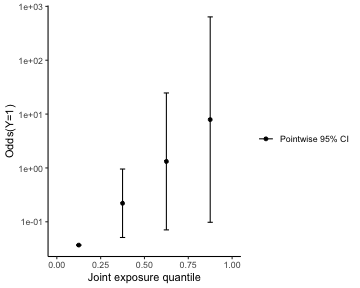Continuous modifiers

Here we simulate some data, similar to prior datasets, where we use a continuous modifier.

set.seed(23)
dat3 <- simdata_quantized_emm(
outcometype="continuous",
# sample size
n = 100,
# correlation between x1 and x2,x3,...
corr=c(0.8,0.6,0.3,-0.3,-0.3,-0.3),
# model intercept
b0=-2,
# linear model coefficients for x1,x2,... at referent level of interacting variable
mainterms=c(0.3,-0.1,0.1,0.0,0.3,0.1,0.1),
# linear model coefficients for product terms between x1,x2,... and interacting variable
prodterms = c(1.0,0.0,0.0,0.0,0.2,0.2,0.2),
# type of interacting variable
ztype = "continuous",
# number of levels of exposure
q = 4,
# residual variance of y
yscale = 2.0
)
names(dat3)[which(names(dat3)=="z")] = "CoM"

##          CoM x1 x2 x3 x4 x5 x6 x7          y
## 1  0.1932123  3  3  3  3  0  0  3 -1.0597465
## 2 -0.4346821  2  2  3  2  0  1  1 -4.9330438
## 3  0.9132671  1  1  0  1  2  2  2 -1.8409107
## 4  1.7933881  1  1  2  1  3  2  0  1.6776239
## 5  0.9966051  2  2  0  2  0  1  1 -1.0562066
## 6  1.1074905  1  1  3  0  2  1  0 -0.9831373
summary(dat3$CoM) ## Min. 1st Qu. Median Mean 3rd Qu. Max. ## -2.41826 -0.53582 0.04267 0.07475 0.84181 1.97606 summary(dat3$y)
##    Min. 1st Qu.  Median    Mean 3rd Qu.    Max.
## -8.6878 -2.7252 -0.8718 -0.7253  1.2805  8.0743
cor(dat3[,paste0("x",1:7)])
##        x1     x2     x3     x4     x5     x6     x7
## x1  1.000  0.920  0.632  0.320 -0.400 -0.520 -0.200
## x2  0.920  1.000  0.616  0.320 -0.400 -0.472 -0.136
## x3  0.632  0.616  1.000  0.232 -0.360 -0.288 -0.240
## x4  0.320  0.320  0.232  1.000 -0.040 -0.280  0.032
## x5 -0.400 -0.400 -0.360 -0.040  1.000  0.192  0.072
## x6 -0.520 -0.472 -0.288 -0.280  0.192  1.000  0.024
## x7 -0.200 -0.136 -0.240  0.032  0.072  0.024  1.000
qfit3 <- qgcomp.emm.noboot(y~x1+x2+x3+x4+x5+x6+x7,
data = dat3,
expnms = paste0("x",1:7),
emmvar = "CoM",
q = 4)
qfit3
## ## Qgcomp weights/partial effects at CoM = 0
## Scaled effect size (positive direction, sum of positive effects = 0.93)
##      x3      x1      x7      x6      x4      x5
## 0.29883 0.24796 0.20602 0.20568 0.03698 0.00453
## Scaled effect size (negative direction, sum of negative effects = -0.482)
## x2
##  1
##
##
## ## Mixture slope parameters (Delta method CI):
##
##             Estimate Std. Error Lower CI Upper CI t value Pr(>|t|)
## (Intercept) -1.40872    0.82889 -3.03332  0.21587 -1.6995  0.09292
## psi1         0.44806    0.54005 -0.61041  1.50654  0.8297  0.40907
## CoM         -1.70779    0.95928 -3.58795  0.17236 -1.7803  0.07864
## CoM:mixture  2.78425    0.62382  1.56160  4.00691  4.4633 2.49e-05
qfit3b <- qgcomp.emm.boot(y~x1+x2+x3+x4+x5+x6+x7,
data = dat3,
expnms = paste0("x",1:7),
emmvar = "CoM",
q = 4)
qfit3b
## ## Mixture slope parameters (bootstrap CI):
##
##             Estimate Std. Error Lower CI Upper CI t value  Pr(>|t|)
## (Intercept) -1.40872    0.94283 -3.25664  0.43920 -1.4941 0.1389319
## psi1         0.44806    0.59199 -0.71221  1.60834  0.7569 0.4512656
## CoM         -1.70779    1.05839 -3.78221  0.36662 -1.6136 0.1104158
## CoM:mixture  2.78425    0.69043  1.43103  4.13748  4.0326 0.0001219

Getting point wise comparisons at specific values of a continuous confounder

Point-wise comparisons are available for non-bootstrapped fits

print("output/plot the weights at CoM=0")
##  "output/plot the weights at CoM=0"
getstratweights(qfit3, emmval=0)
## ## Qgcomp weights/partial effects at CoM = 0
## Scaled effect size (positive direction, sum of positive effects = 0.93)
##      x3      x1      x7      x6      x4      x5
## 0.29883 0.24796 0.20602 0.20568 0.03698 0.00453
## Scaled effect size (negative direction, sum of negative effects = -0.482)
## x2
##  1
plot(qfit3, emmval=0)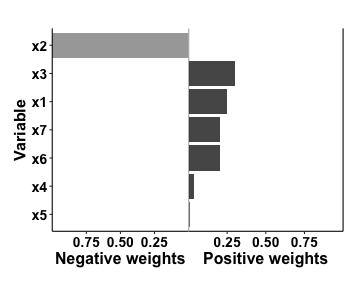print("output stratum specific joint effect estimate for the mixture at CoM=0")
##  "output stratum specific joint effect estimate for the mixture at CoM=0"
print(getstrateffects(qfit3, emmval=0))
## Joint effect at CoM=0
##         Estimate Std. Error Lower CI Upper CI z value Pr(>|z|)
## Mixture  0.44806    0.54005 -0.61041   1.5065  0.8297   0.4067
print("output pointwise comparisons at CoM=0")
##  "output pointwise comparisons at CoM=0"
print(pointwisebound(qfit3, emmval=0))
##   quantile quantile.midpoint          hx mean.diff   se.diff    ll.diff
## 1        0             0.125 -1.40872323 0.0000000 0.0000000  0.0000000
## 2        1             0.375 -0.96065965 0.4480636 0.5400468 -0.6104086
## 3        2             0.625 -0.51259608 0.8961271 1.0800935 -1.2208173
## 4        3             0.875 -0.06453251 1.3441907 1.6201403 -1.8312259
##    ul.diff emm_level
## 1 0.000000         0
## 2 1.506536         0
## 3 3.013072         0
## 4 4.519607         0
print("output/plot the weights at the 80%ile of CoM")
##  "output/plot the weights at the 80%ile of CoM"
getstratweights(qfit3, emmval=quantile(dat3$CoM, .8)) ## ## Qgcomp weights/partial effects at CoM = 0.975896138278307 ## Scaled effect size (positive direction, sum of positive effects = 3.18) ## x1 x7 x6 x5 x2 x3 ## 0.2402 0.2321 0.2212 0.1308 0.0934 0.0822 ## Scaled effect size (negative direction, sum of negative effects = -0.0196) ## x4 ## 1 plot(qfit3, emmval=quantile(dat3$CoM, .8))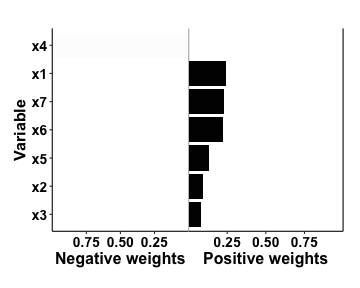print("output stratum specific joint effect estimate for the mixture at the 80%ile of CoM")
##  "output stratum specific joint effect estimate for the mixture at the 80%ile of CoM"
print(getstrateffects(qfit3, emmval=quantile(dat3$CoM, .8))) ## Joint effect at CoM=0.975896138278307 ## Estimate Std. Error Lower CI Upper CI z value Pr(>|z|) ## Mixture 3.16521 0.80046 1.5963 4.7341 3.9542 7.678e-05 print("output pointwise comparisons at at the 80%ile of CoM") ##  "output pointwise comparisons at at the 80%ile of CoM" print(pointwisebound(qfit3, emmval=quantile(dat3$CoM, .8)))
##   quantile quantile.midpoint         hx mean.diff   se.diff  ll.diff   ul.diff
## 1        0             0.125 -3.0753528  0.000000 0.0000000 0.000000  0.000000
## 2        1             0.375  0.0898543  3.165207 0.8004573 1.596340  4.734075
## 3        2             0.625  3.2550614  6.330414 1.6009147 3.192679  9.468149
## 4        3             0.875  6.4202685  9.495621 2.4013720 4.789019 14.202224
##   emm_level
## 1 0.9758961
## 2 0.9758961
## 3 0.9758961
## 4 0.9758961

Point-wise comparisons are variably available for bootstrapped fits (work in progress)

print("plot the pointwise effects at CoM=0")
##  "plot the pointwise effects at CoM=0"
plot(qfit3b, emmval=0)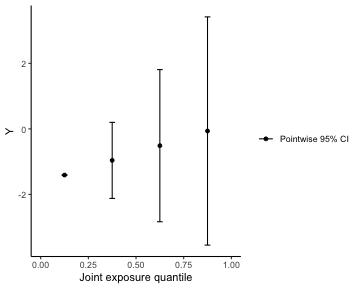print("output stratum specific joint effect estimate for the mixture at CoM=0")
##  "output stratum specific joint effect estimate for the mixture at CoM=0"
print(getstrateffects(qfit3b, emmval=0))
## Joint effect at CoM=0
##         Estimate Std. Error Lower CI Upper CI z value Pr(>|z|)
## Mixture  0.44806    0.54005 -0.61041   1.5065  0.8297   0.4067
print("output pointwise comparisons at CoM=0")
##  "output pointwise comparisons at CoM=0"
print(pointwisebound(qfit3b, emmval=0))
##   quantile quantile.midpoint          hx mean.diff   se.diff    ll.diff
## 1        0             0.125 -1.40872323 0.0000000 0.0000000  0.0000000
## 2        1             0.375 -0.96065965 0.4480636 0.5919884 -0.7122124
## 3        2             0.625 -0.51259608 0.8961271 1.1839768 -1.4244248
## 4        3             0.875 -0.06453251 1.3441907 1.7759653 -2.1366372
##    ul.diff ll.linpred ul.linpred emm_level
## 1 0.000000  -1.408723 -1.4087232         0
## 2 1.608340  -2.120936  0.1996163         0
## 3 3.216679  -2.833148  1.8079559         0
## 4 4.825019  -3.545360  3.4162954         0
print("plot the pointwise effects at the 80%ile of CoM")
##  "plot the pointwise effects at the 80%ile of CoM"
plot(qfit3b, emmval=quantile(dat3$CoM, .8))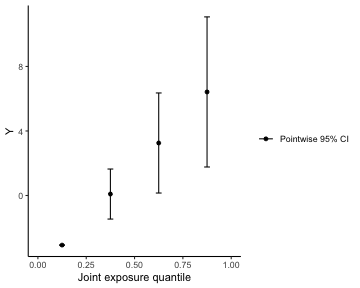print("output stratum specific joint effect estimate for the mixture at the 80%ile of CoM") ##  "output stratum specific joint effect estimate for the mixture at the 80%ile of CoM" print(getstrateffects(qfit3b, emmval=quantile(dat3$CoM, .8)))
## Joint effect at CoM=0.975896138278307
##         Estimate Std. Error Lower CI Upper CI z value  Pr(>|z|)
## Mixture  3.16521    0.80046   1.5963   4.7341  3.9542 7.678e-05
print("output pointwise comparisons at at the 80%ile of CoM")
##  "output pointwise comparisons at at the 80%ile of CoM"
print(pointwisebound(qfit3b, emmval=quantile(dat3$CoM, .8))) ## quantile quantile.midpoint hx mean.diff se.diff ll.diff ul.diff ## 1 0 0.125 -3.0753528 0.000000 0.0000000 0.000000 0.000000 ## 2 1 0.375 0.0898543 3.165207 0.7913566 1.614177 4.716237 ## 3 2 0.625 3.2550614 6.330414 1.5827131 3.228353 9.432475 ## 4 3 0.875 6.4202685 9.495621 2.3740697 4.842530 14.148712 ## ll.linpred ul.linpred emm_level ## 1 -3.0753528 -3.075353 0.9758961 ## 2 -1.4611761 1.640885 0.9758961 ## 3 0.1530007 6.357122 0.9758961 ## 4 1.7671774 11.073360 0.9758961 Non-linear fits Non-linear joint effects of a mixture will tend to occur when independent effects of individual exposures are non-linear or when there is interaction on the model scale between exposures. Here is a toy example of allowing a quadratic overall joint effect with an underlying set of interaction terms between x1 and all other exposures. q is set to 8 for this example for reasons described in the qgcomp package vignette. This approach adds an interaction term for both the “main effect” of the mixture and the squared term of the mixture. The coefficients can be intererpreted as any other quadratic/interaction terms (which is to say, with some difficulty). Informally, the CoM:mixture2 term can be interpreted as the magnitude of the change in the non-linearity of the slope due to a one unit increase in the continuous modifier. qfit3bnl <- qgcomp.emm.boot(y~x1+x2+x3+x4+x5+x6+x7 + x1*(x2 + x3 + x4 + x5 + x6 +x7), data = dat3, expnms = paste0("x",1:7), emmvar = "CoM", q = 8, degree= 2) qfit3bnl ## ## Mixture slope parameters (bootstrap CI): ## ## Estimate Std. Error Lower CI Upper CI t value Pr(>|t|) ## (Intercept) -1.8359e+00 1.2208e+00 -4.2286e+00 5.5678e-01 -1.5039 0.1367573 ## psi1 4.5330e-01 1.0235e+00 -1.5527e+00 2.4593e+00 0.4429 0.6590888 ## psi2 -6.6350e-02 2.2012e-01 -4.9778e-01 3.6508e-01 -0.3014 0.7639148 ## CoM -1.1514e+00 9.2798e-01 -2.9702e+00 6.6743e-01 -1.2407 0.2185184 ## CoM:mixture 1.6314e+00 4.0471e-01 8.3815e-01 2.4246e+00 4.0309 0.0001308 ## CoM:mixture^2 1.0240e-16 2.1799e-16 -3.2485e-16 5.2965e-16 0.4697 0.6398895 Some effect estimation tools are not all enabled for non-linear fits and will produce an error. However, as with previous fits, we can estimate pointwise differences along the quantiles, and plot the marginal structural model regression line at various values of the joint quantized exposures. Here you can see that the regression line is expected to be steeper at higher levels of the modifier, as in previous fits. We can explictly ask for model confidence bands (which are given by the modelbound function), which pull in confidence limits for the regression line based on the bootstrap distribution of estimates. print(pointwisebound(qfit3bnl, emmval=-1)) ## quantile quantile.midpoint hx mean.diff se.diff ll.diff ## 1 0 0.0625 -0.6845197 0.000000 0.0000000 0.000000 ## 2 1 0.1875 -1.9289318 -1.244412 0.9562061 -3.118542 ## 3 2 0.3125 -3.3060446 -2.621525 1.5901647 -5.738190 ## 4 3 0.4375 -4.8158581 -4.131338 2.0054956 -8.062038 ## 5 4 0.5625 -6.4583724 -5.773853 2.3951061 -10.468174 ## 6 5 0.6875 -8.2335875 -7.549068 3.0293769 -13.486537 ## 7 6 0.8125 -10.1415032 -9.456983 4.1245156 -17.540886 ## 8 7 0.9375 -12.1821197 -11.497600 5.7507213 -22.768807 ## ul.diff ll.linpred ul.linpred emm_level ## 1 0.0000000 -0.6845197 -0.6845197 -1 ## 2 0.6297175 -3.8030614 -0.0548022 -1 ## 3 0.4951406 -6.4227101 -0.1893791 -1 ## 4 -0.2006392 -8.7465573 -0.8851589 -1 ## 5 -1.0795311 -11.1526940 -1.7640508 -1 ## 6 -1.6115982 -14.1710570 -2.2961179 -1 ## 7 -1.3730814 -18.2254053 -2.0576011 -1 ## 8 -0.2263934 -23.4533263 -0.9109132 -1 print(pointwisebound(qfit3bnl, emmval=-1)) ## quantile quantile.midpoint hx mean.diff se.diff ll.diff ## 1 0 0.0625 -0.6845197 0.000000 0.0000000 0.000000 ## 2 1 0.1875 -1.9289318 -1.244412 0.9562061 -3.118542 ## 3 2 0.3125 -3.3060446 -2.621525 1.5901647 -5.738190 ## 4 3 0.4375 -4.8158581 -4.131338 2.0054956 -8.062038 ## 5 4 0.5625 -6.4583724 -5.773853 2.3951061 -10.468174 ## 6 5 0.6875 -8.2335875 -7.549068 3.0293769 -13.486537 ## 7 6 0.8125 -10.1415032 -9.456983 4.1245156 -17.540886 ## 8 7 0.9375 -12.1821197 -11.497600 5.7507213 -22.768807 ## ul.diff ll.linpred ul.linpred emm_level ## 1 0.0000000 -0.6845197 -0.6845197 -1 ## 2 0.6297175 -3.8030614 -0.0548022 -1 ## 3 0.4951406 -6.4227101 -0.1893791 -1 ## 4 -0.2006392 -8.7465573 -0.8851589 -1 ## 5 -1.0795311 -11.1526940 -1.7640508 -1 ## 6 -1.6115982 -14.1710570 -2.2961179 -1 ## 7 -1.3730814 -18.2254053 -2.0576011 -1 ## 8 -0.2263934 -23.4533263 -0.9109132 -1 print(pointwisebound(qfit3bnl, emmval=1)) ## quantile quantile.midpoint hx mean.diff se.diff ll.diff ul.diff ## 1 0 0.0625 -2.9872820 0.000000 0.000000 0.0000000 0.000000 ## 2 1 0.1875 -0.9689631 2.018319 0.887790 0.2782826 3.758355 ## 3 2 0.3125 0.9166552 3.903937 1.475282 1.0124375 6.795437 ## 4 3 0.4375 2.6695727 5.656855 1.890157 1.9522141 9.361495 ## 5 4 0.5625 4.2897894 7.277071 2.355340 2.6606898 11.893453 ## 6 5 0.6875 5.7773054 8.764587 3.136064 2.6180157 14.911159 ## 7 6 0.8125 7.1321206 10.119403 4.396709 1.5020116 18.736794 ## 8 7 0.9375 8.3542352 11.341517 6.172158 -0.7556912 23.438725 ## ll.linpred ul.linpred emm_level ## 1 -2.9872820 -2.9872820 1 ## 2 -2.7089994 0.7710733 1 ## 3 -1.9748445 3.8081549 1 ## 4 -1.0350680 6.3742133 1 ## 5 -0.3265922 8.9061710 1 ## 6 -0.3692664 11.9238771 1 ## 7 -1.4852704 15.7495117 1 ## 8 -3.7429732 20.4514435 1 plot(qfit3bnl, emmval=-1, modelband=TRUE, pointwiseref=4)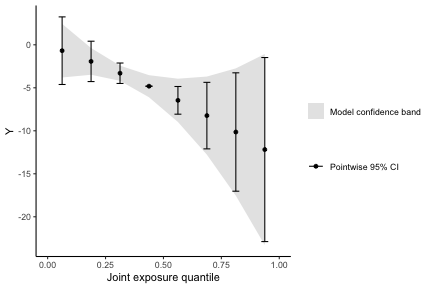plot(qfit3bnl, emmval=1, modelband=TRUE, pointwiseref=4)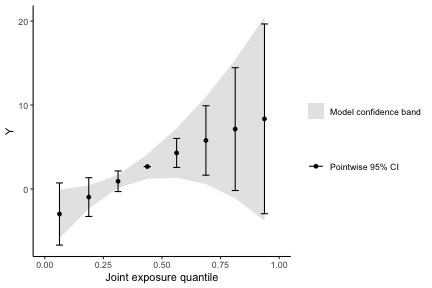We can look at this fit another way, too, by plotting predictions from the marginal structural model at all observed values of the modifier, which gives a more complete picture of the model than the plots at single values of the modifier. We can create smoothed scatter plot lines (LOESS) at binned values of the modifier to informally look at how the regression line might change over values of the modifier. We can approximate the effect (point estimate only) at a specific value of z by plotting a smooth fit limited a narrow range of the modifier (< -1 or > 1). Here we see that the joint effect of exposures does appear to differ across values of the modifier, but there is little suggestion of non-linearity at either low or high values of the modifier. This informal assessment agrees with intuition based on the estimated coefficients and the standard plots from the qgcompint package. library(ggplot2) plotdata = data=data.frame(q=qfit3bnl$index, ey=qfit3bnl$y.expected, modifier=qfit3bnl$emmvar.msm)
ggplot() +
geom_point(aes(x=q, y=ey, color=modifier), data=plotdata) +
geom_point(aes(x=q, y=ey), color="purple", data=plotdata[plotdata$modifier>1,], pch=1, cex=3) + geom_smooth(aes(x=q, y=ey), se=FALSE, color="purple", data=plotdata[plotdata$modifier>1,], method = 'loess', formula='y ~ x') +
geom_smooth(aes(x=q, y=ey), se=FALSE, color="red", data=plotdata[plotdata$modifier < -1,], method = 'loess', formula='y ~ x') + geom_point(aes(x=q, y=ey), color="red", data=plotdata[plotdata$modifier < -1,], pch=1, cex=3) +
theme_classic() +
labs(y="Expected outcome", x="Quantile score value (0 to q-1)") +
scale_color_continuous(name="Value\nof\nmodifier")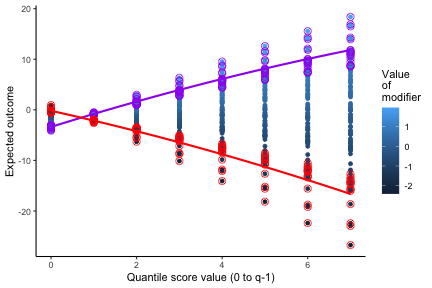Survival analysis

As with standard qgcomp, the qgcompint package allows assessment of effect measure modification for a Cox proportional hazards model. The simdata_quantized_emm function allows simulation of right censored survival data.

set.seed(23)
dat4 <- simdata_quantized_emm(
outcometype="survival",
# sample size
n = 200,
# correlation between x1 and x2,x3,...
corr=c(0.8,0.6,0.3,-0.3,-0.3,-0.3),
# model intercept
b0=-2,
# linear model coefficients for x1,x2,... at referent level of interacting variable
mainterms=c(0.0,-0.1,0.1,0.0,0.3,0.1,0.1),
# linear model coefficients for product terms between x1,x2,... and interacting variable
prodterms = c(0.1,0.0,0.0,0.0,-0.2,-0.2,-0.2),
# type of interacting variable
ztype = "categorical",
# number of levels of exposure
q = 4,
# residual variance of y
yscale = 2.0
)
dat4$zfactor = as.factor(dat4$z)
##   z x1 x2 x3 x4 x5 x6 x7     time d zfactor
## 1 0  3  3  3  2  0  0  0 2.544399 1       0
## 2 2  3  3  3  3  3  2  0 3.419209 1       2
## 3 0  2  2  1  2  3  1  1 1.775714 1       0
## 4 2  3  3  3  3  3  3  3 4.000000 0       2
## 5 2  0  0  0  0  3  1  3 3.329749 1       2
## 6 0  0  0  0  0  1  2  3 1.557125 1       0
summary(dat4$zfactor) ## 0 1 2 ## 64 63 73 summary(dat4$time)
##    Min. 1st Qu.  Median    Mean 3rd Qu.    Max.
##  0.4161  1.9309  2.6441  2.6728  3.7167  4.0000
table(dat4\$d) # 30 censored
##
##   0   1
##  40 160
cor(dat4[,paste0("x",1:7)])
##        x1     x2     x3     x4     x5     x6     x7
## x1  1.000  0.776  0.616  0.268 -0.296 -0.328 -0.268
## x2  0.776  1.000  0.468  0.192 -0.200 -0.316 -0.284
## x3  0.616  0.468  1.000  0.228 -0.272 -0.244 -0.284
## x4  0.268  0.192  0.228  1.000 -0.064  0.056 -0.176
## x5 -0.296 -0.200 -0.272 -0.064  1.000  0.032  0.220
## x6 -0.328 -0.316 -0.244  0.056  0.032  1.000  0.004
## x7 -0.268 -0.284 -0.284 -0.176  0.220  0.004  1.000

Fitting a Cox model with a fully linear/additive specification is very similar to other qgcomp “noboot” models, and the same plots/weight estimation/effect estimation functions work on these objects. For now, bootstrapped versions of this model are not available.

qfit4 <- qgcomp.emm.cox.noboot(survival::Surv(time, d)~x1+x2+x3+x4+x5+x6+x7,
data = dat4,
expnms = paste0("x",1:7),
emmvar = "zfactor",
q = 4)
## Adding main term for zfactor to the model
qfit4
## ## Qgcomp weights/partial effects at zfactor = 0
## Scaled effect size (positive direction, sum of positive effects = 0.773)
##     x7     x6     x5     x4     x3
## 0.3457 0.3062 0.1686 0.1063 0.0732
## Scaled effect size (negative direction, sum of negative effects = -0.142)
##     x2     x1
## 0.9686 0.0314
##
##
## Mixture log(hazard ratio) (Delta method CI):
##
##                   Estimate Std. Error  Lower CI Upper CI Z value Pr(>|z|)
## psi1              0.630742   0.351180 -0.057559  1.31904  1.7961  0.07248
## zfactor1         -0.465127   0.809987 -2.052673  1.12242 -0.5742  0.56580
## zfactor1:mixture -0.060184   0.519398 -1.078185  0.95782 -0.1159  0.90775
## zfactor2          0.746395   0.797326 -0.816334  2.30913  0.9361  0.34921
## zfactor2:mixture -1.325227   0.525392 -2.354976 -0.29548 -2.5224  0.01166
plot(qfit4, emmval=0)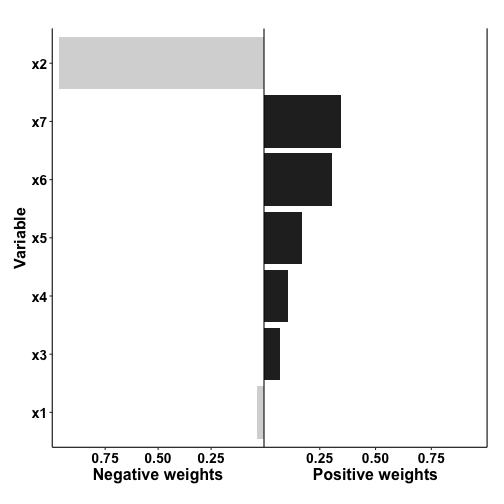getstratweights(qfit4, emmval=2)
## ## Qgcomp weights/partial effects at zfactor = 2
## Scaled effect size (positive direction, sum of positive effects = 0.421)
##   x3   x2
## 0.83 0.17
## Scaled effect size (negative direction, sum of negative effects = -1.12)
##     x6     x1     x7     x5     x4
## 0.3468 0.2427 0.2276 0.1250 0.0578
getstrateffects(qfit4, emmval=2)
## Joint effect at zfactor=2
##         Estimate Std. Error Lower CI Upper CI z value Pr(>|z|)
## Mixture -0.69448    0.38722  -1.4534 0.064445 -1.7935  0.07289
pointwisebound(qfit4, emmval=1)
##   quantile quantile.midpoint         hx       hr   se.lnhr     ll.hr    ul.hr
## 1        0             0.125 -0.4651275 1.000000 0.0000000 1.0000000  1.00000
## 2        1             0.375  0.1054305 1.769254 0.3891288 0.8252075  3.79330
## 3        2             0.625  0.6759885 3.130260 0.7782575 0.6809675 14.38912
## 4        3             0.875  1.2465464 5.538224 1.1673863 0.5619395 54.58226
##   emm_level
## 1         1
## 2         1
## 3         1
## 4         1

Multiple modifiers

There is currently no simple way to implement multiple, simultaneous modifiers in the qgcompint package. For binary/categorical modifiers, it is straightforward to create a single modifier with distinct value for every unique combination of the modifiers.